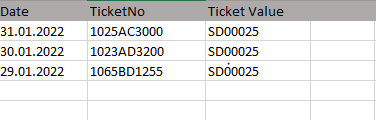# How to get the distinct value from a column of a datatable in string format

I am trying to get the distinct value from an column in an string format, I have been using it within a loop. Each time when getting the distinct value, i use to have an single value only.

Approach which i have used is finding the unique data in a datatable format and then converting it to string using output datatable activity.dt1 = dt_Main.DefaultView.ToTable(true, “Ticket Value”)

followed by output datatable activity

where i convert the datatable variable to string.

I am suppose to use the final string value into SAP. Using the above process i am getting the value in string but each time i use the string value in Type into activity, it is used along with Enter hotkey.

Need suggestion on how to get the distinct value in string format, as i have an single value to find from an column.

1 Like

Hi!

can we try like this:

For each row in data table the in value:

yourDT.DefaultView.ToTable(true, “ColumnName”)

if you wants to convert the dt variable to string we have an activity called output data table which gives you the string output

Regards,
NaNi

@THIRU_NANI , Thanks for the response, I have already tried the way as i mentioned above, but not getting the exact output, as using the above process when using the output into Type into activity, The input is copied along with Enter, which i don’t want to have, Please suggest if there is any way where i can get the output directly into the string format.

Hi!

Why we need to convert that in to string? if we have to enter that value to the SAP simply read the distinct values from excel then take type into and indicate it on SAP Platform and pass the variable.

Regards,
NaNi

We can get the Distinct Value from a Column of a Datatable using the `Distinct()` method.

However, we need to note that if there are more than two different values we get two distinct values as the output.

Could you try with the below Expression and Let us know if it is the Output that was Expected :

``````DT.AsEnumerable.Select(Function(x)x("Ticket Value").ToString).Distinct.First
``````

Hi

You are almost done

Just mention like this to get the distinct value as a string from datatable

str_input = dt_Main.DefaultView.ToTable(true, “Ticket Value”).Rows(0)(0).ToString

This will get first row - first column - cell value as a string